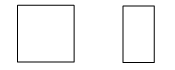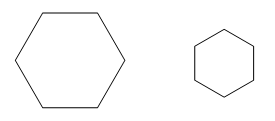# Similarity

Similar figures have the same shape, but not necessarily the same size. Two plane figures are called similar if one can be enlarged so that it is congruent to the other figure.

For shapes to be similar they must satisfy both the following conditions:

• matching angles are equal
• matching sides are proportional.

These two rectangles have matching angles equal but their matching sides are not in the same ratio and so they are not similar.Non-similar rectangles.

These two hexagons have matching sides in the same proportion and their matching angles are equal and so they are similar.Similar regular hexagons.

All congruent shapes are similar because all matching angles are equal and the matching sides are in proportion (in the ratio 1:1).

Investigate further the effect of the changing proportion of sides for similar shapes with the learning object L11103: Using a centre of enlargement.

Watch the video Non-similar and Similar Triangles.

## Similar triangles

Pairs of triangles are similar if they meet the conditions of any one of four standard tests.

Once proven to be similar, matching angles will be equal and matching sides will be proportional.